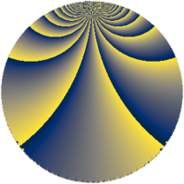# Properties

 Label 405.4.rLevel $405$ Weight $4$ Character orbit 405.r Rep. character $\chi_{405}(8,\cdot)$ Character field $\Q(\zeta_{36})$ Dimension $624$ Sturm bound $216$

# Related objects

## Defining parameters

 Level: $$N$$ $$=$$ $$405 = 3^{4} \cdot 5$$ Weight: $$k$$ $$=$$ $$4$$ Character orbit: $$[\chi]$$ $$=$$ 405.r (of order $$36$$ and degree $$12$$) Character conductor: $$\operatorname{cond}(\chi)$$ $$=$$ $$135$$ Character field: $$\Q(\zeta_{36})$$ Sturm bound: $$216$$

## Dimensions

The following table gives the dimensions of various subspaces of $$M_{4}(405, [\chi])$$.

Total New Old
Modular forms 2016 672 1344
Cusp forms 1872 624 1248
Eisenstein series 144 48 96

## Trace form

 $$624 q + 12 q^{2} + 12 q^{5} - 12 q^{7} + 18 q^{8} + O(q^{10})$$ $$624 q + 12 q^{2} + 12 q^{5} - 12 q^{7} + 18 q^{8} - 6 q^{10} - 12 q^{13} - 24 q^{16} + 18 q^{17} - 756 q^{20} - 12 q^{22} + 324 q^{23} + 420 q^{25} - 24 q^{28} - 24 q^{31} - 1752 q^{32} - 2466 q^{35} - 6 q^{37} + 132 q^{38} - 396 q^{40} - 1680 q^{41} - 12 q^{43} - 12 q^{46} + 3480 q^{47} + 684 q^{50} + 84 q^{52} - 24 q^{55} + 4752 q^{56} - 12 q^{58} - 132 q^{61} + 18 q^{62} - 2076 q^{65} + 3660 q^{67} - 2676 q^{68} - 12 q^{70} + 36 q^{71} - 6 q^{73} - 792 q^{76} + 3324 q^{77} - 24 q^{82} + 2832 q^{83} - 12 q^{85} + 12516 q^{86} - 3036 q^{88} - 12 q^{91} + 1938 q^{92} + 4302 q^{95} + 6900 q^{97} + 5832 q^{98} + O(q^{100})$$

## Decomposition of $$S_{4}^{\mathrm{new}}(405, [\chi])$$ into newform subspaces

The newforms in this space have not yet been added to the LMFDB.

## Decomposition of $$S_{4}^{\mathrm{old}}(405, [\chi])$$ into lower level spaces

$$S_{4}^{\mathrm{old}}(405, [\chi]) \cong$$ $$S_{4}^{\mathrm{new}}(135, [\chi])$$$$^{\oplus 2}$$